# RD Sharma Solutions For Class 7 Maths Exercise 5.2 Chapter 5 Operations On Rational Numbers

RD Sharma Solutions for Class 7 Exercise 5.2 of Chapter 5 Operations on Rational Numbers is the best study material for students whose aim is to excel in board exams. The PDF of RD Sharma Solutions for Class 7 Exercise 5.2 are provided here. The students having confusion while solving RD Sharma questions can clear their doubts as the RD Sharma solutions for class 7 is given here for the chapter Operations on Rational Numbers. This exercise includes the topic of subtraction of rational numbers. Here students will also learn about the additive inverse of rational numbers.

## Download the PDF of RD Sharma Solutions For Class 7 Maths Chapter 5 – Operations On Rational Numbers Exercise 5.2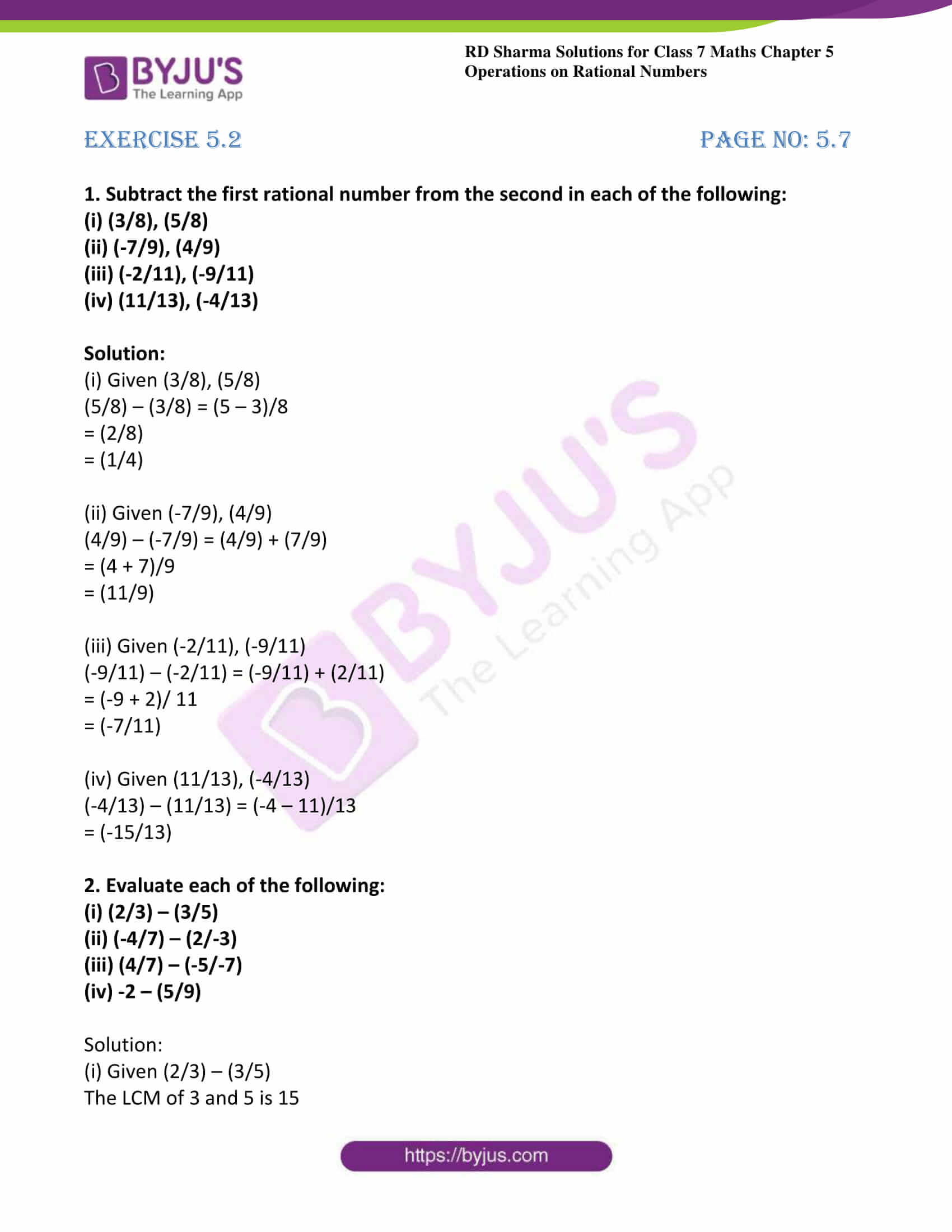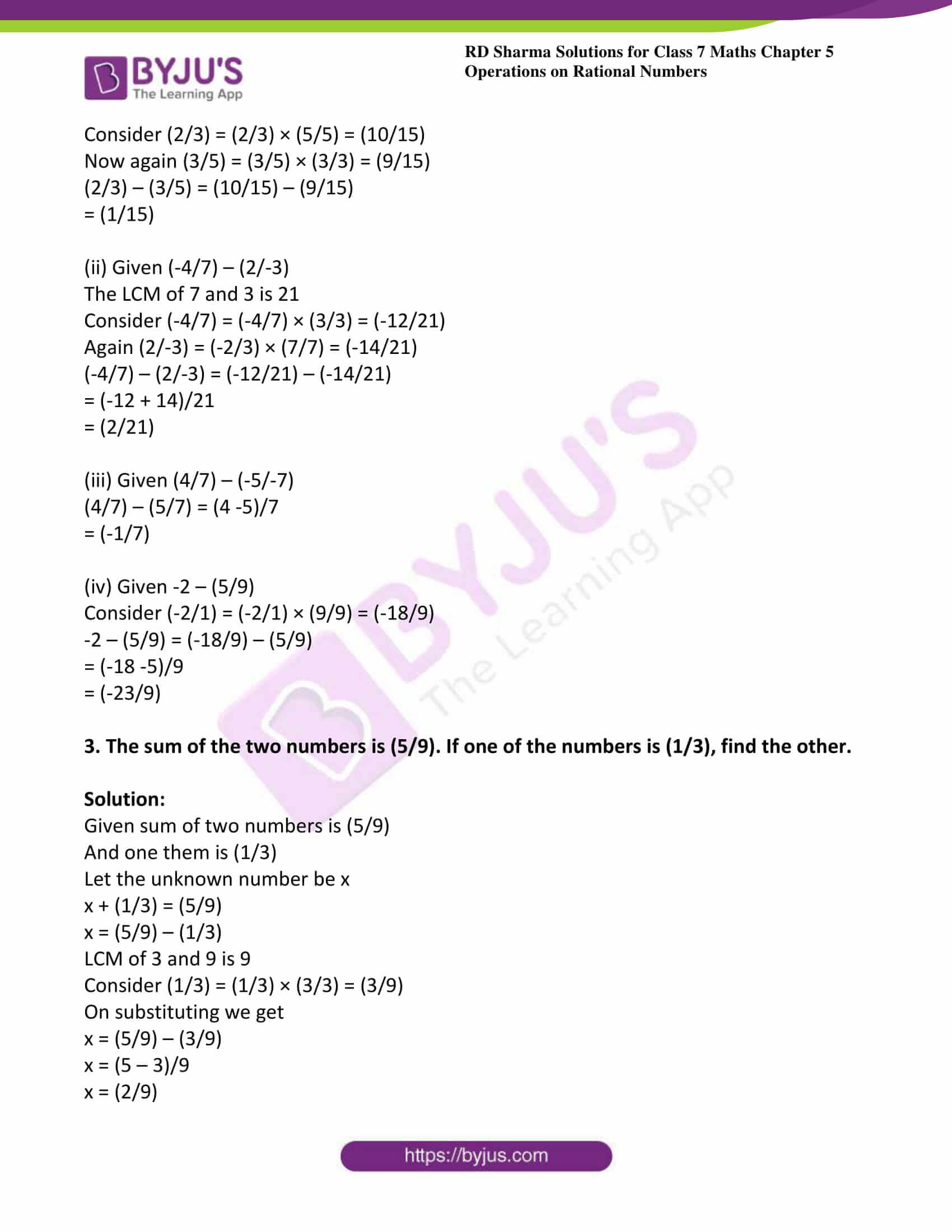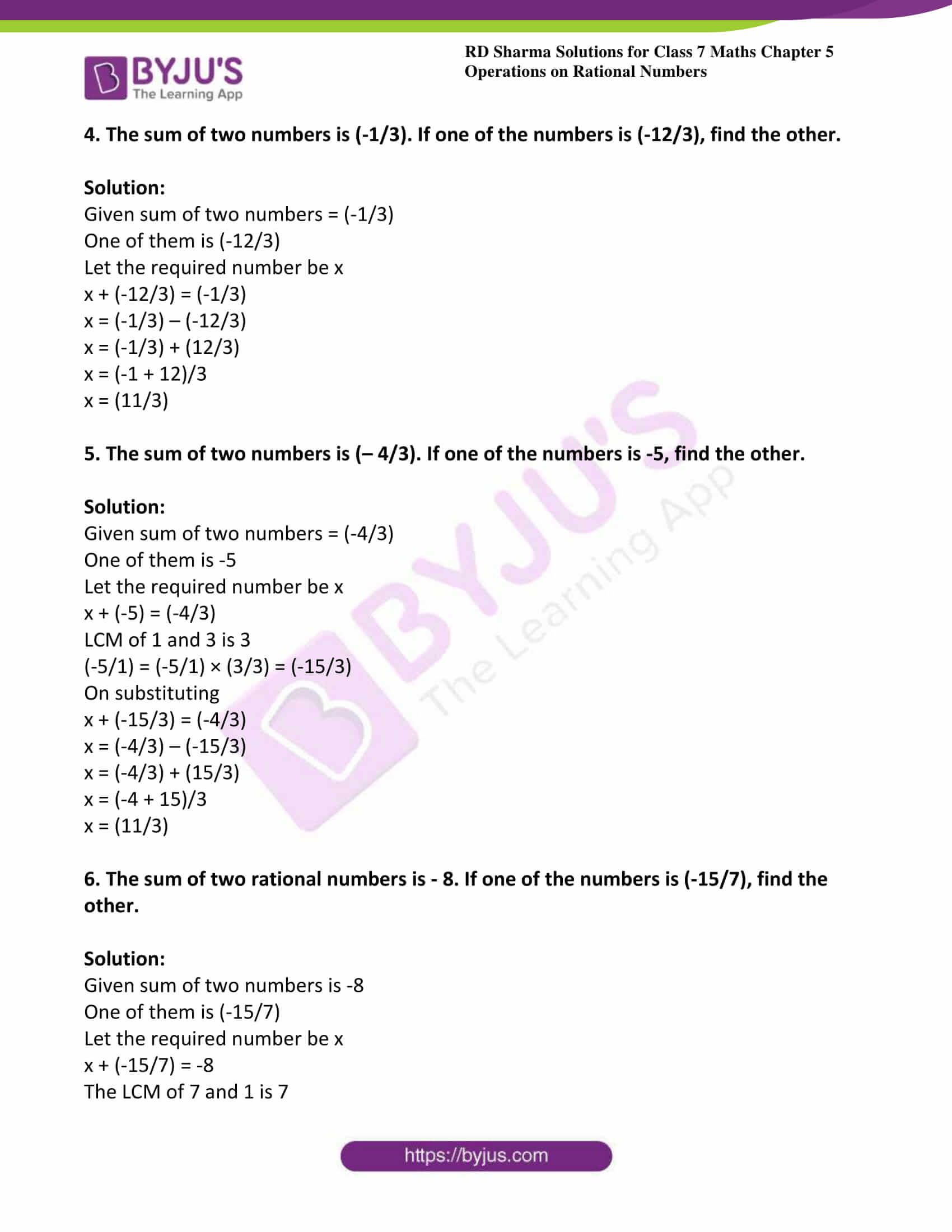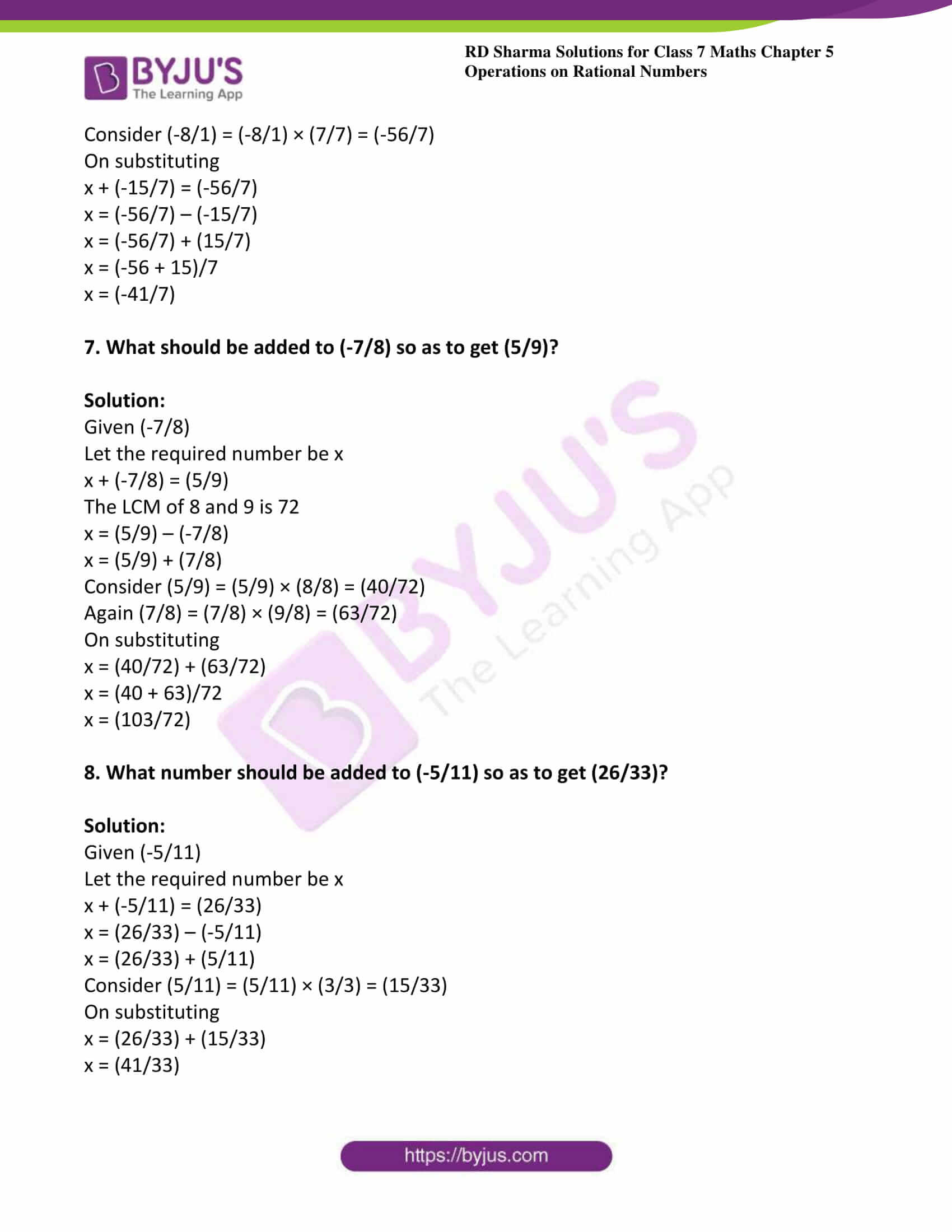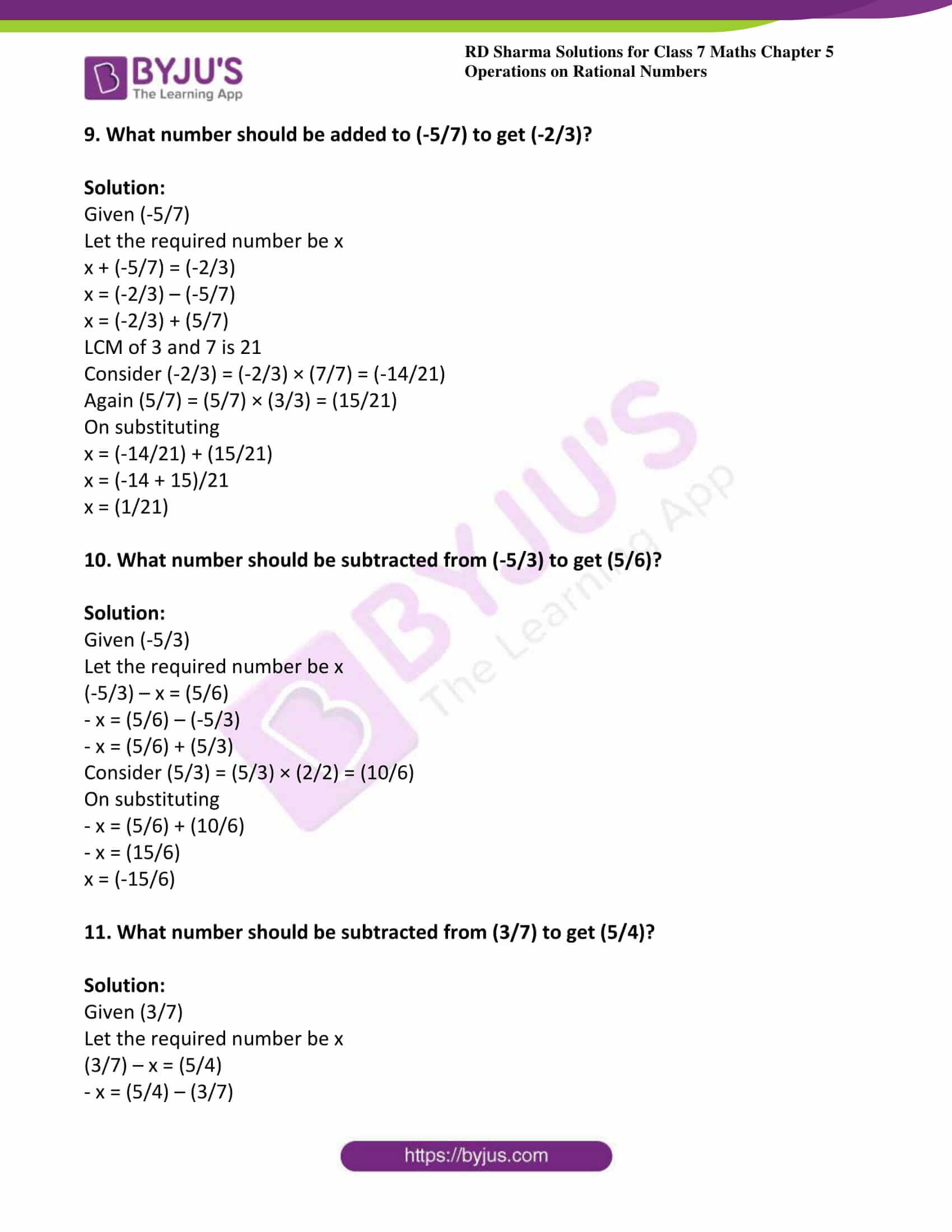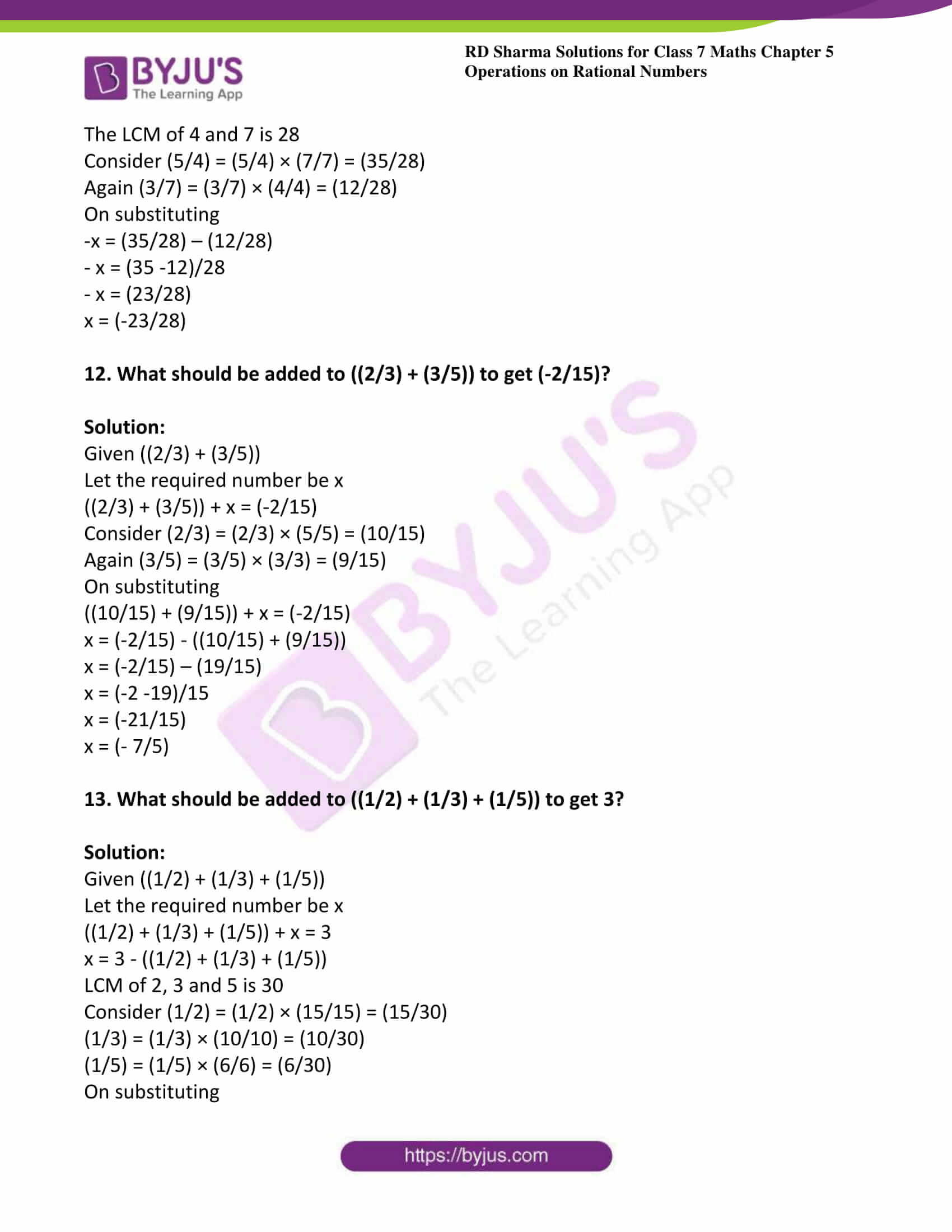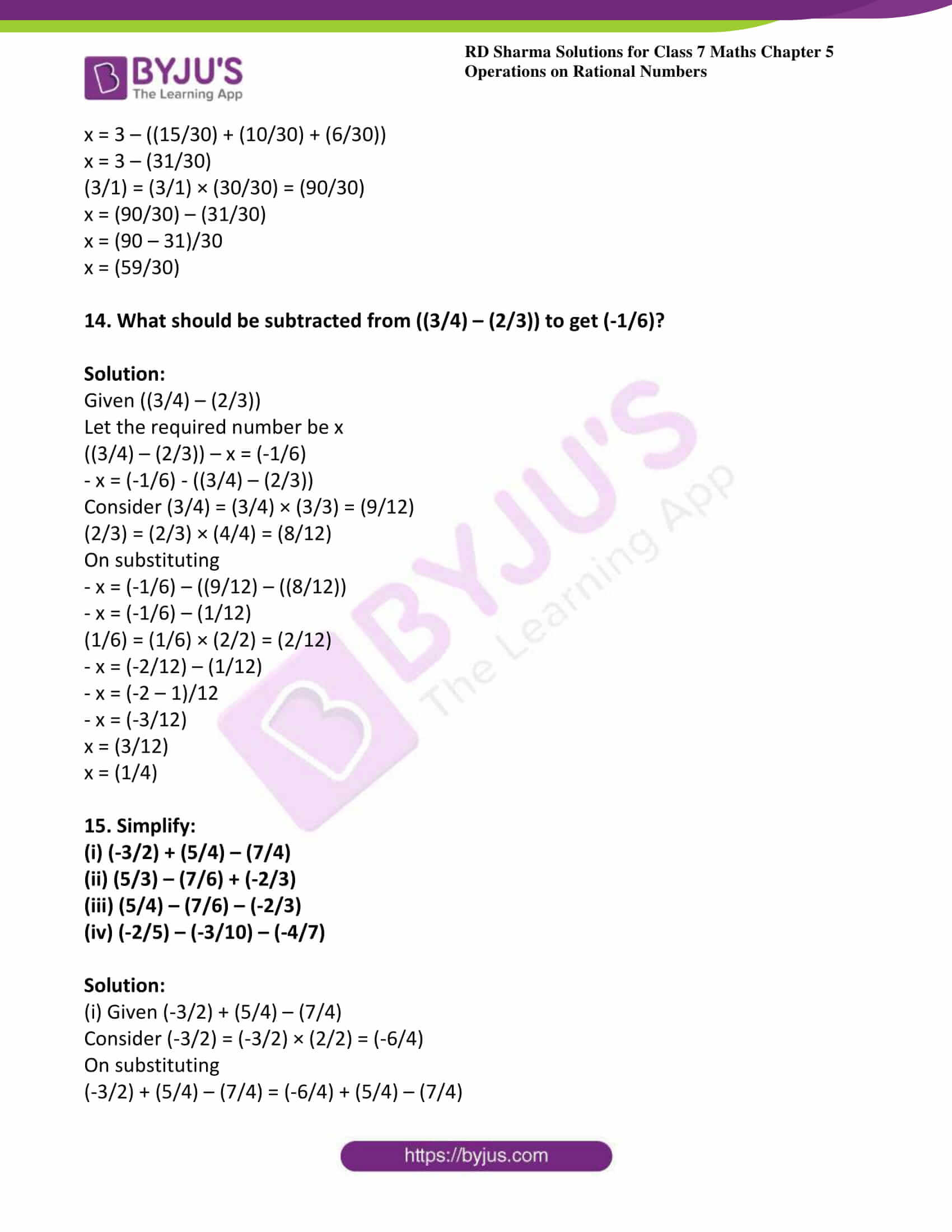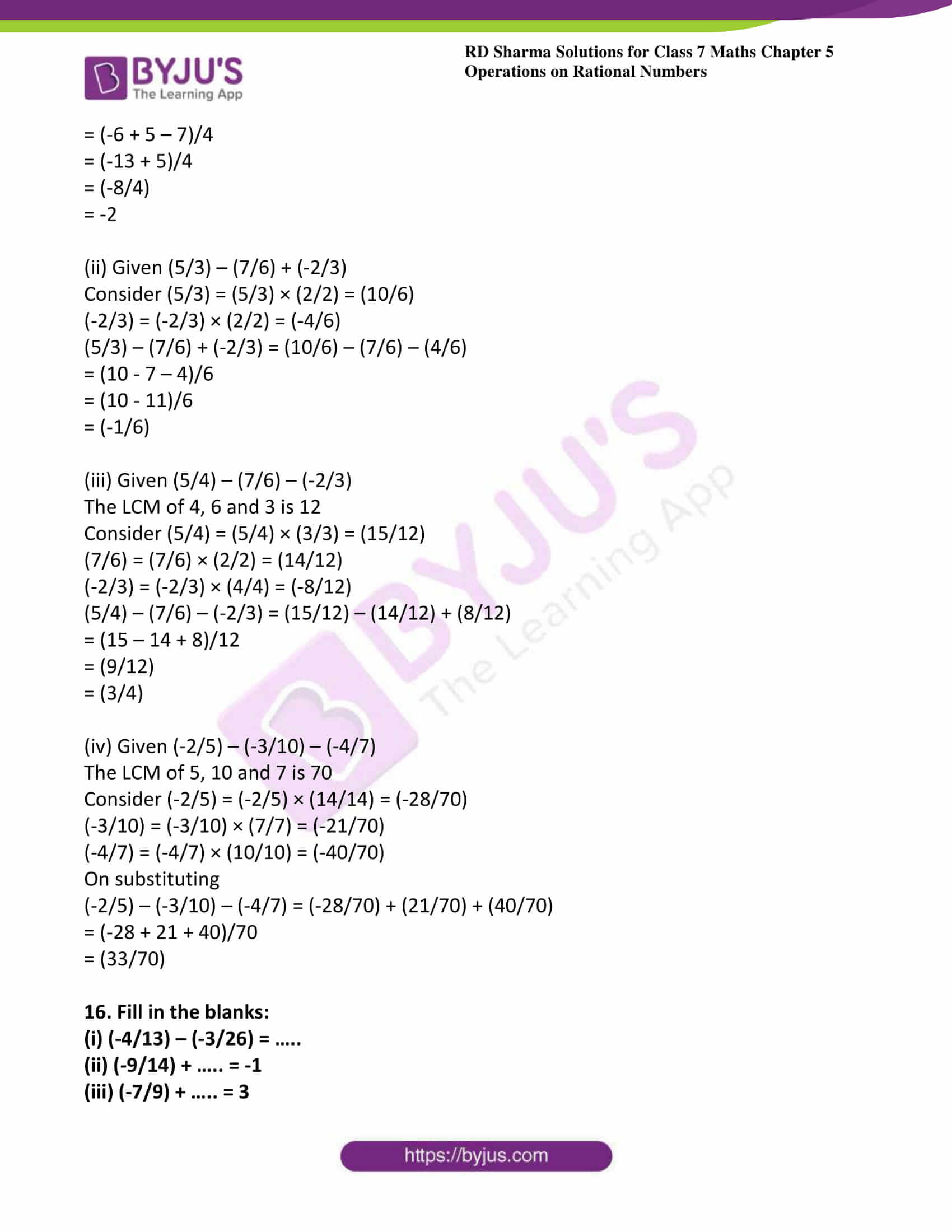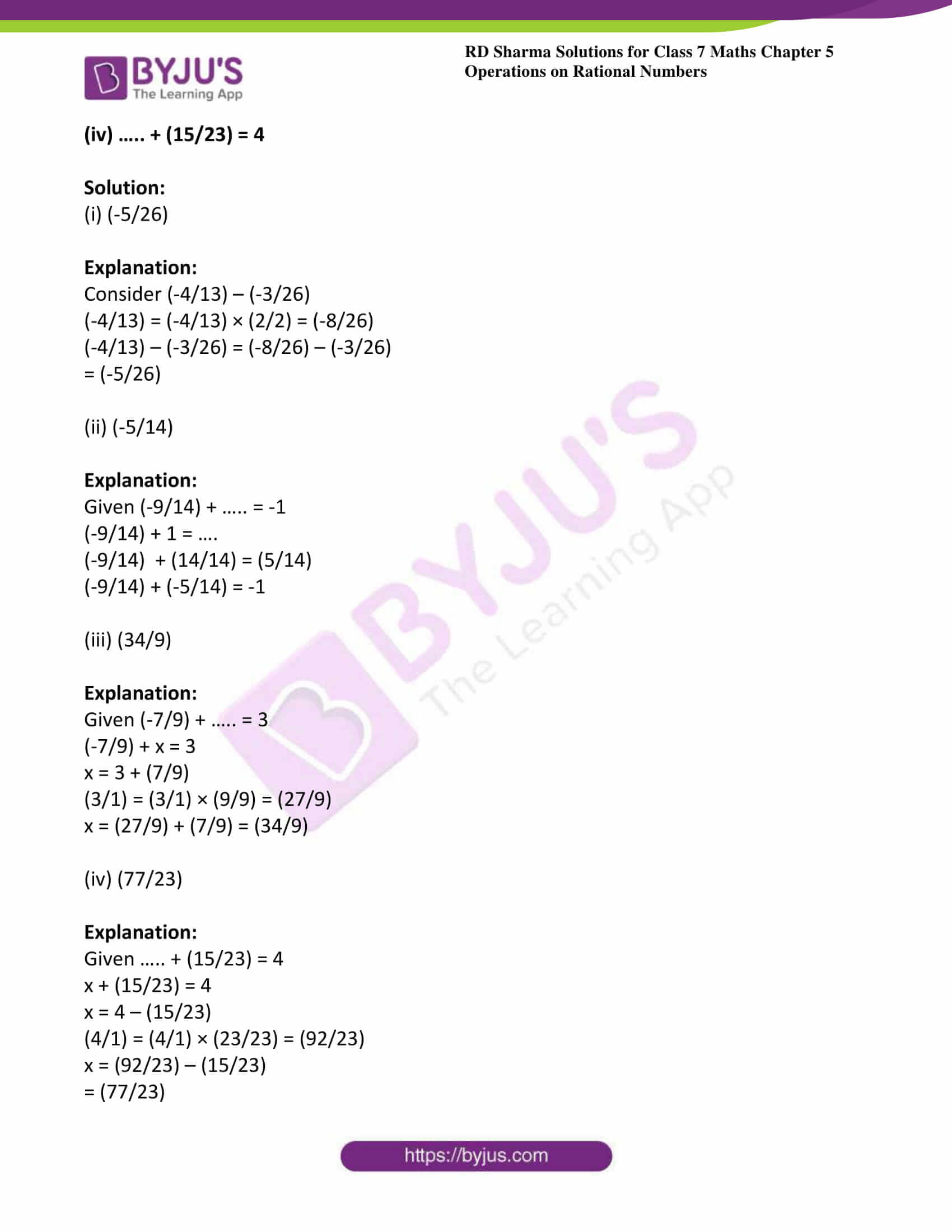### Access answers to Maths RD Sharma Solutions For Class 7 Chapter 5 – Operations On Rational Numbers Exercise 5.2

1. Subtract the first rational number from the second in each of the following:

(i) (3/8), (5/8)

(ii) (-7/9), (4/9)

(iii) (-2/11), (-9/11)

(iv) (11/13), (-4/13)

Solution:

(i) Given (3/8), (5/8)

(5/8) – (3/8) = (5 – 3)/8

= (2/8)

= (1/4)

(ii) Given (-7/9), (4/9)

(4/9) – (-7/9) = (4/9) + (7/9)

= (4 + 7)/9

= (11/9)

(iii) Given (-2/11), (-9/11)

(-9/11) – (-2/11) = (-9/11) + (2/11)

= (-9 + 2)/ 11

= (-7/11)

(iv) Given (11/13), (-4/13)

(-4/13) – (11/13) = (-4 – 11)/13

= (-15/13)

2. Evaluate each of the following:

(i) (2/3) – (3/5)

(ii) (-4/7) – (2/-3)

(iii) (4/7) – (-5/-7)

(iv) -2 – (5/9)

Solution:

(i) Given (2/3) – (3/5)

The LCM of 3 and 5 is 15

Consider (2/3) = (2/3) × (5/5) = (10/15)

Now again (3/5) = (3/5) × (3/3) = (9/15)

(2/3) – (3/5) = (10/15) – (9/15)

= (1/15)

(ii) Given (-4/7) – (2/-3)

The LCM of 7 and 3 is 21

Consider (-4/7) = (-4/7) × (3/3) = (-12/21)

Again (2/-3) = (-2/3) × (7/7) = (-14/21)

(-4/7) – (2/-3) = (-12/21) – (-14/21)

= (-12 + 14)/21

= (2/21)

(iii) Given (4/7) – (-5/-7)

(4/7) – (5/7) = (4 -5)/7

= (-1/7)

(iv) Given -2 – (5/9)

Consider (-2/1) = (-2/1) × (9/9) = (-18/9)

-2 – (5/9) = (-18/9) – (5/9)

= (-18 -5)/9

= (-23/9)

3. The sum of the two numbers is (5/9). If one of the numbers is (1/3), find the other.

Solution:

Given sum of two numbers is (5/9)

And one them is (1/3)

Let the unknown number be x

x + (1/3) = (5/9)

x = (5/9) – (1/3)

LCM of 3 and 9 is 9

Consider (1/3) = (1/3) × (3/3) = (3/9)

On substituting we get

x = (5/9) – (3/9)

x = (5 – 3)/9

x = (2/9)

4. The sum of two numbers is (-1/3). If one of the numbers is (-12/3), find the other.

Solution:

Given sum of two numbers = (-1/3)

One of them is (-12/3)

Let the required number be x

x + (-12/3) = (-1/3)

x = (-1/3) – (-12/3)

x = (-1/3) + (12/3)

x = (-1 + 12)/3

x = (11/3)

5. The sum of two numbers is (– 4/3). If one of the numbers is -5, find the other.

Solution:

Given sum of two numbers = (-4/3)

One of them is -5

Let the required number be x

x + (-5) = (-4/3)

LCM of 1 and 3 is 3

(-5/1) = (-5/1) × (3/3) = (-15/3)

On substituting

x + (-15/3) = (-4/3)

x = (-4/3) – (-15/3)

x = (-4/3) + (15/3)

x = (-4 + 15)/3

x = (11/3)

6. The sum of two rational numbers is – 8. If one of the numbers is (-15/7), find the other.

Solution:

Given sum of two numbers is -8

One of them is (-15/7)

Let the required number be x

x + (-15/7) = -8

The LCM of 7 and 1 is 7

Consider (-8/1) = (-8/1) × (7/7) = (-56/7)

On substituting

x + (-15/7) = (-56/7)

x = (-56/7) – (-15/7)

x = (-56/7) + (15/7)

x = (-56 + 15)/7

x = (-41/7)

7. What should be added to (-7/8) so as to get (5/9)?

Solution:

Given (-7/8)

Let the required number be x

x + (-7/8) = (5/9)

The LCM of 8 and 9 is 72

x = (5/9) – (-7/8)

x = (5/9) + (7/8)

Consider (5/9) = (5/9) × (8/8) = (40/72)

Again (7/8) = (7/8) × (9/8) = (63/72)

On substituting

x = (40/72) + (63/72)

x = (40 + 63)/72

x = (103/72)

8. What number should be added to (-5/11) so as to get (26/33)?

Solution:

Given (-5/11)

Let the required number be x

x + (-5/11) = (26/33)

x = (26/33) – (-5/11)

x = (26/33) + (5/11)

Consider (5/11) = (5/11) × (3/3) = (15/33)

On substituting

x = (26/33) + (15/33)

x = (41/33)

9. What number should be added to (-5/7) to get (-2/3)?

Solution:

Given (-5/7)

Let the required number be x

x + (-5/7) = (-2/3)

x = (-2/3) – (-5/7)

x = (-2/3) + (5/7)

LCM of 3 and 7 is 21

Consider (-2/3) = (-2/3) × (7/7) = (-14/21)

Again (5/7) = (5/7) × (3/3) = (15/21)

On substituting

x = (-14/21) + (15/21)

x = (-14 + 15)/21

x = (1/21)

10. What number should be subtracted from (-5/3) to get (5/6)?

Solution:

Given (-5/3)

Let the required number be x

(-5/3) – x = (5/6)

– x = (5/6) – (-5/3)

– x = (5/6) + (5/3)

Consider (5/3) = (5/3) × (2/2) = (10/6)

On substituting

– x = (5/6) + (10/6)

– x = (15/6)

x = (-15/6)

11. What number should be subtracted from (3/7) to get (5/4)?

Solution:

Given (3/7)

Let the required number be x

(3/7) – x = (5/4)

– x = (5/4) – (3/7)

The LCM of 4 and 7 is 28

Consider (5/4) = (5/4) × (7/7) = (35/28)

Again (3/7) = (3/7) × (4/4) = (12/28)

On substituting

-x = (35/28) – (12/28)

– x = (35 -12)/28

– x = (23/28)

x = (-23/28)

12. What should be added to ((2/3) + (3/5)) to get (-2/15)?

Solution:

Given ((2/3) + (3/5))

Let the required number be x

((2/3) + (3/5)) + x = (-2/15)

Consider (2/3) = (2/3) × (5/5) = (10/15)

Again (3/5) = (3/5) × (3/3) = (9/15)

On substituting

((10/15) + (9/15)) + x = (-2/15)

x = (-2/15) – ((10/15) + (9/15))

x = (-2/15) – (19/15)

x = (-2 -19)/15

x = (-21/15)

x = (- 7/5)

13. What should be added to ((1/2) + (1/3) + (1/5)) to get 3?

Solution:

Given ((1/2) + (1/3) + (1/5))

Let the required number be x

((1/2) + (1/3) + (1/5)) + x = 3

x = 3 – ((1/2) + (1/3) + (1/5))

LCM of 2, 3 and 5 is 30

Consider (1/2) = (1/2) × (15/15) = (15/30)

(1/3) = (1/3) × (10/10) = (10/30)

(1/5) = (1/5) × (6/6) = (6/30)

On substituting

x = 3 – ((15/30) + (10/30) + (6/30))

x = 3 – (31/30)

(3/1) = (3/1) × (30/30) = (90/30)

x = (90/30) – (31/30)

x = (90 – 31)/30

x = (59/30)

14. What should be subtracted from ((3/4) – (2/3)) to get (-1/6)?

Solution:

Given ((3/4) – (2/3))

Let the required number be x

((3/4) – (2/3)) – x = (-1/6)

– x = (-1/6) – ((3/4) – (2/3))

Consider (3/4) = (3/4) × (3/3) = (9/12)

(2/3) = (2/3) × (4/4) = (8/12)

On substituting

– x = (-1/6) – ((9/12) – ((8/12))

– x = (-1/6) – (1/12)

(1/6) = (1/6) × (2/2) = (2/12)

– x = (-2/12) – (1/12)

– x = (-2 – 1)/12

– x = (-3/12)

x = (3/12)

x = (1/4)

15. Simplify:

(i) (-3/2) + (5/4) – (7/4)

(ii) (5/3) – (7/6) + (-2/3)

(iii) (5/4) – (7/6) – (-2/3)

(iv) (-2/5) – (-3/10) – (-4/7)

Solution:

(i) Given (-3/2) + (5/4) – (7/4)

Consider (-3/2) = (-3/2) × (2/2) = (-6/4)

On substituting

(-3/2) + (5/4) – (7/4) = (-6/4) + (5/4) – (7/4)

= (-6 + 5 – 7)/4

= (-13 + 5)/4

= (-8/4)

= -2

(ii) Given (5/3) – (7/6) + (-2/3)

Consider (5/3) = (5/3) × (2/2) = (10/6)

(-2/3) = (-2/3) × (2/2) = (-4/6)

(5/3) – (7/6) + (-2/3) = (10/6) – (7/6) – (4/6)

= (10 – 7 – 4)/6

= (10 – 11)/6

= (-1/6)

(iii) Given (5/4) – (7/6) – (-2/3)

The LCM of 4, 6 and 3 is 12

Consider (5/4) = (5/4) × (3/3) = (15/12)

(7/6) = (7/6) × (2/2) = (14/12)

(-2/3) = (-2/3) × (4/4) = (-8/12)

(5/4) – (7/6) – (-2/3) = (15/12) – (14/12) + (8/12)

= (15 – 14 + 8)/12

= (9/12)

= (3/4)

(iv) Given (-2/5) – (-3/10) – (-4/7)

The LCM of 5, 10 and 7 is 70

Consider (-2/5) = (-2/5) × (14/14) = (-28/70)

(-3/10) = (-3/10) × (7/7) = (-21/70)

(-4/7) = (-4/7) × (10/10) = (-40/70)

On substituting

(-2/5) – (-3/10) – (-4/7) = (-28/70) + (21/70) + (40/70)

= (-28 + 21 + 40)/70

= (33/70)

16. Fill in the blanks:

(i) (-4/13) – (-3/26) = …..

(ii) (-9/14) + ….. = -1

(iii) (-7/9) + ….. = 3

(iv) ….. + (15/23) = 4

Solution:

(i) (-5/26)

Explanation:

Consider (-4/13) – (-3/26)

(-4/13) = (-4/13) × (2/2) = (-8/26)

(-4/13) – (-3/26) = (-8/26) – (-3/26)

= (-5/26)

(ii) (-5/14)

Explanation:

Given (-9/14) + ….. = -1

(-9/14) + 1 = ….

(-9/14) + (14/14) = (5/14)

(-9/14) + (-5/14) = -1

(iii) (34/9)

Explanation:

Given (-7/9) + ….. = 3

(-7/9) + x = 3

x = 3 + (7/9)

(3/1) = (3/1) × (9/9) = (27/9)

x = (27/9) + (7/9) = (34/9)

(iv) (77/23)

Explanation:

Given ….. + (15/23) = 4

x + (15/23) = 4

x = 4 – (15/23)

(4/1) = (4/1) × (23/23) = (92/23)

x = (92/23) – (15/23)

= (77/23)